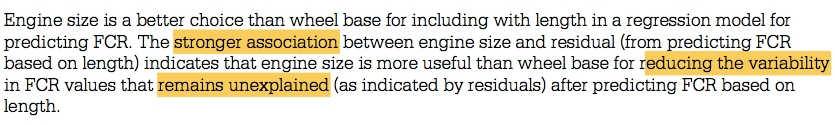# Question 2 (a)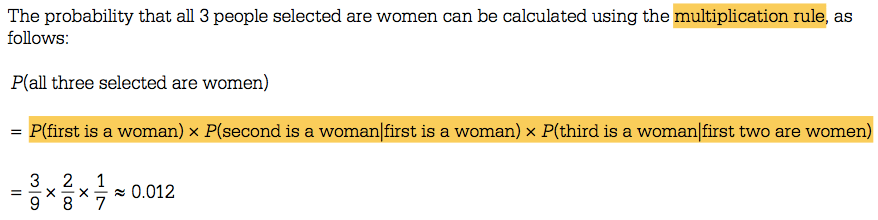# Question 2 (c)# Question 3 (c)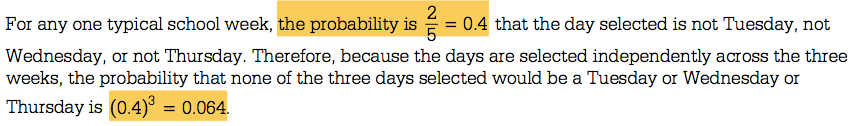# Question 4 (a)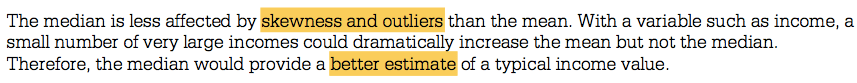# Question 5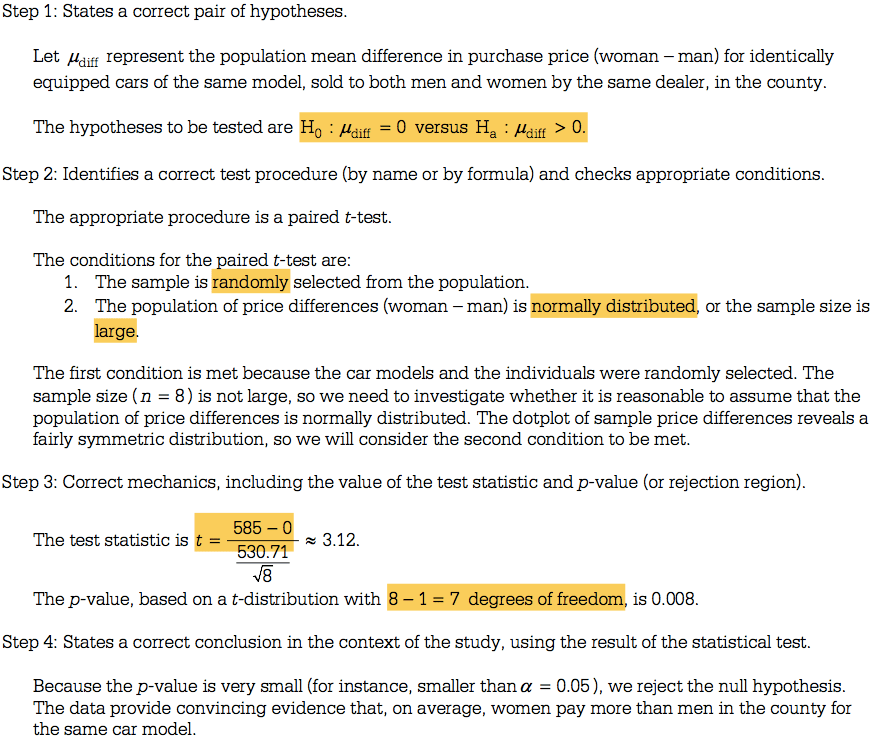• Assumption in 2 sample independence T-test

• Normality: Assumes that the population distributions are normal. The t-test is quite robust over moderate violations of this assumption. It is especially robust if a two tailed test is used and if the sample sizes are not especially small. Check for normality by creating a histogram.

• Independent Observations: The observations within each treatment condition must be independent.

• Equal Variances: Assume that the population distributions have the same variance. This assumption is quite important (If it is violated, it makes the test’s averaging of the 2 variances meaningless).

• If it is violated, then use a modification of the t-test procedures as needed.

• Paired Sample T test

• The matched-pair t-test (or paired t-test or paired samples t-test or dependent t-test) is used when the data from the two groups can be presented in pairs, For example where the same people are being measured in before-and-after comparison or when the group is given two different tests at different times (e.g. pleasantness of two different types of chocolate).
• Assumptions in paired sample t-test

• The first assumption in the paired sample t-test is that only the matched pair can be used to perform the paired sample t-test.

• In the paired sample t-test, normal distributions are assumed.

• Variance in paired sample t-test: in a paired sample t- test, it is assumed that the variance of two sample is same.

• The data is measurement data-interval/ratio

• Independence of observation in paired sample t-test: in a paired sample t-test, observations must be independent of each other.

• Paired t-test vs two-sample t-test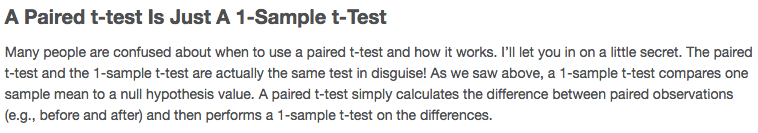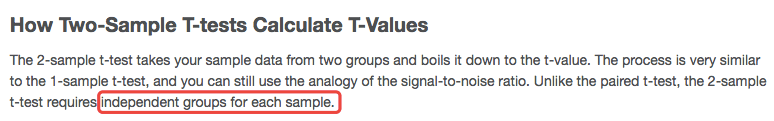• Paired t- test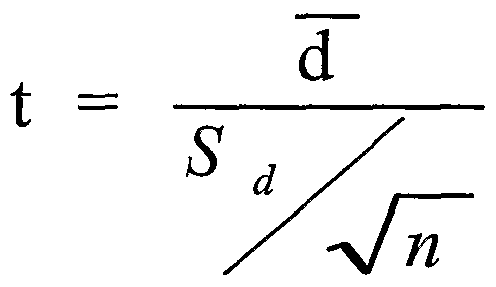# Question 6 (b)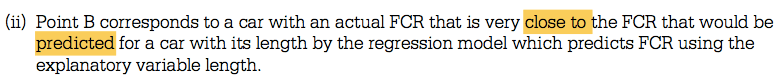# Question 6 (c)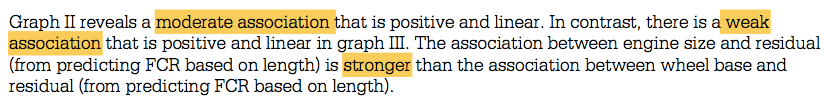# Question 6 (d)